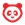cancel
Showing results for
Did you mean:

# How do I weight the final course grade based on assignment groups?

## How do I weight the final course grade based on assignment groups?

You can weight final grades based on assignment groups. Selecting this option assigns a weight to each assignment group, not the assignments themselves. Within each assignment group, a percentage is calculated by dividing the total points a student has earned by the total points possible for all assignments in that group.

For example, if an assignment group included three assignments totaling 25 points, and a student's scores totaled 15 points, the student would earn 60% for the assignment group (15/25). This percentage is then multiplied by the selected group weight. Each assignment group calculation is added together to create the final grade.

For example, an instructor may create three assignment groups (A, B, and C) weighted at 20%, 50%, and 30%, respectively. The total score equation for a course with three assignment groups would be (percentage A x weight A) + (percentage B x weight B) + (percentage C x weight C) = final course percentage. If a student scores 75% in Group A, 98% in Group B, and 87% in Group C, the final score would be calculated as (.75 x .20) + (.98 x .50) + (.87 x .30) = .901, or 90.1%.

The final score calculation is changed if there are no graded items in an assignment group. In this case, all assignment groups with graded items will be divided by their combined weight, and the assignment groups without graded items are removed from the equation. If the previous example were adjusted so Group C contained no graded discussions, assignments, or quizzes, the calculation for final score would be [(.75 x .20) + (.98 x .50)] ÷ .70 = .9143, or 91.43%.

If you choose to use weighted assignment groups, separate assignment groups should be created for each grading period in the course. If an assignment group contains assignments that fall into multiple grading periods with different weighted percentages, grades may have unintended consequences.

Note: If an assignment group is weighted to zero percent, any course items added to the group will not count toward the final grade.

## Open Assignments## Open Assignment Groups Weights SettingsClick the Assignments Options icon  and select the Assignment Groups Weights option .Click the Weight final grade based on assignment groups checkbox.

## Set WeightsEnter the percentage weights for each of the different Assignment Groups you created . The percentage weights you specify here will determine how Canvas calculates the final grade for your course. These percentages can be changed at any time and students will be notified when any changes are made. The weights will show up in the Gradebook for both students and instructors.

Click the Save button .

Notes:

• Assignment group weights can include decimals.
• The total percentage of all assignment groups can be set above or below 100%.
Version history
Revision #:
12 of 12
Last update:
yesterday
Updated by: